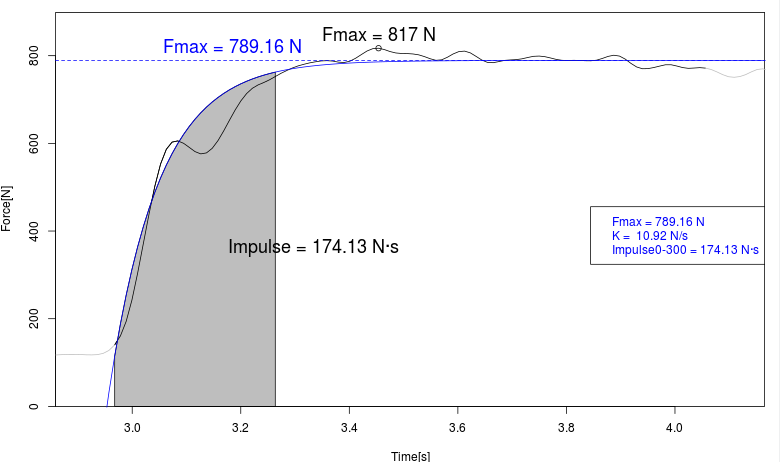# Force tests

Features Results
Isometric force test Evolution of real and modeled force over timeMaximum isometric test illustration:
Maximum isometric force Maximum real and modeled force
RFD (Ratio Force Development) RFD (bounded at defined times, maximum,% Fmax)
Users can define the temporality for the calculation of the Impulse Impulse (Newtons * sec) performed

#### Maximum isometric test illustration: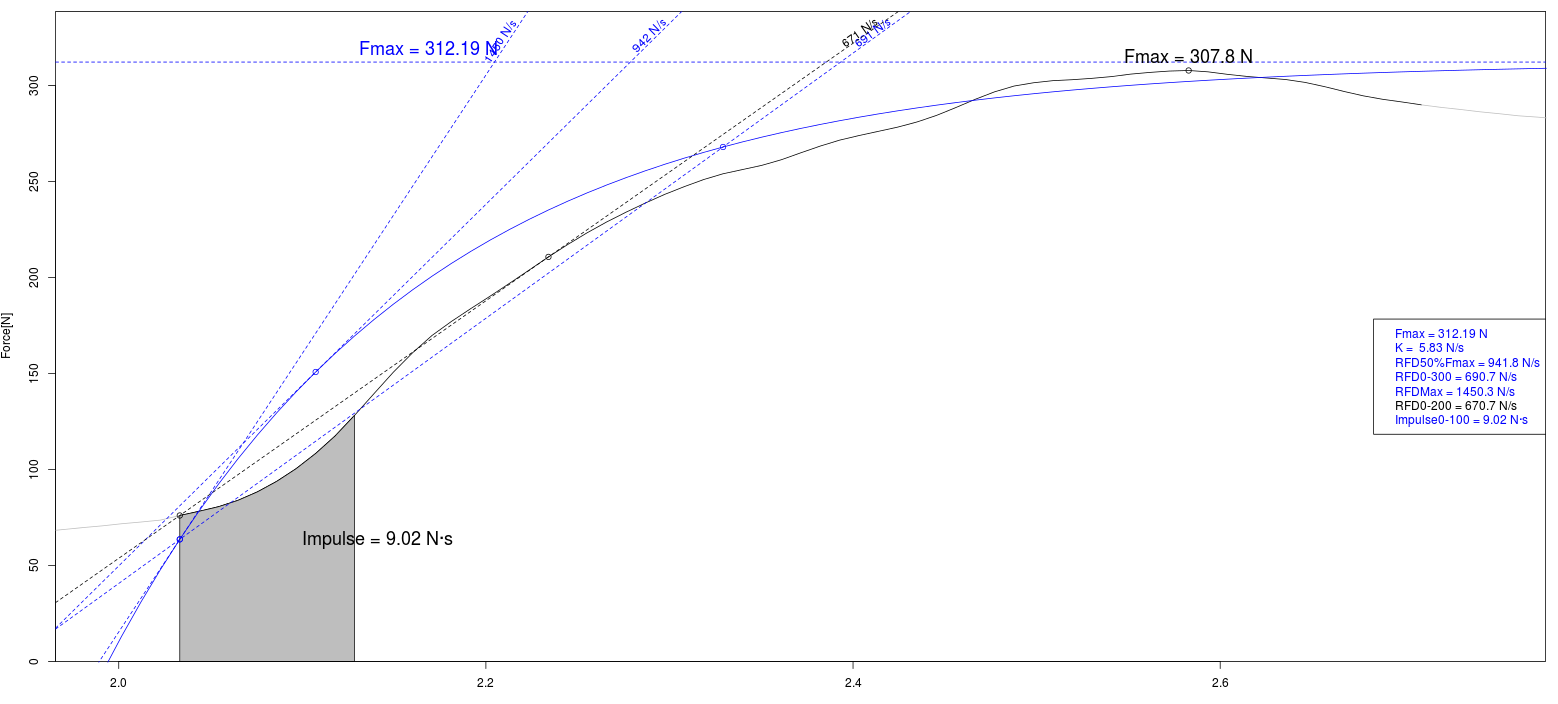Graphics with raw data
Average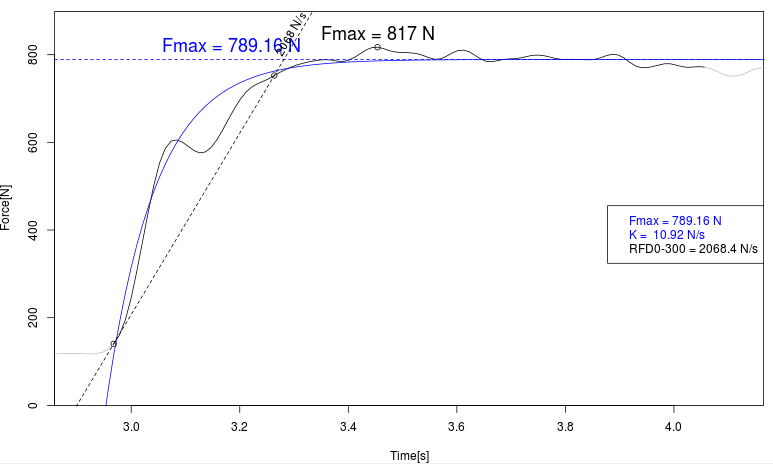Instant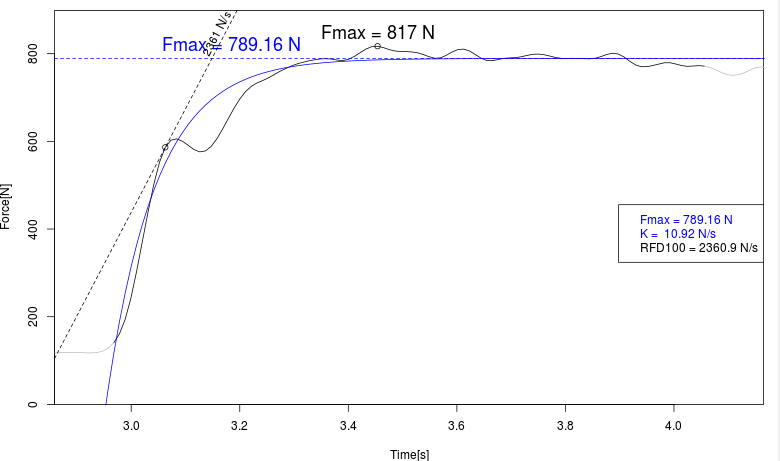% Fmax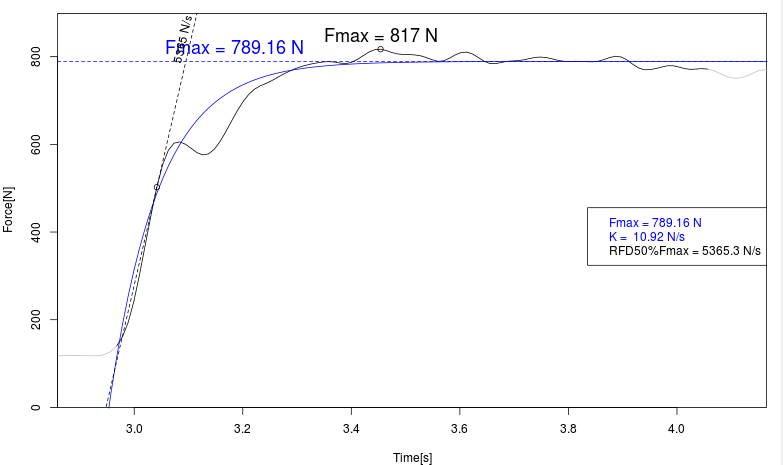Max RFD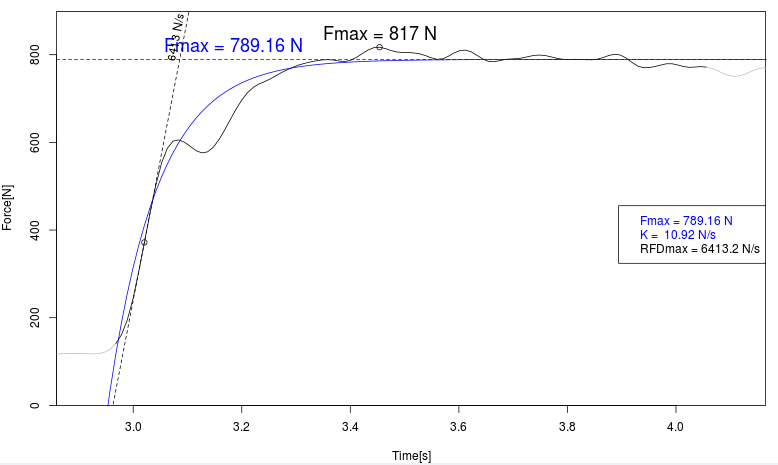Impulse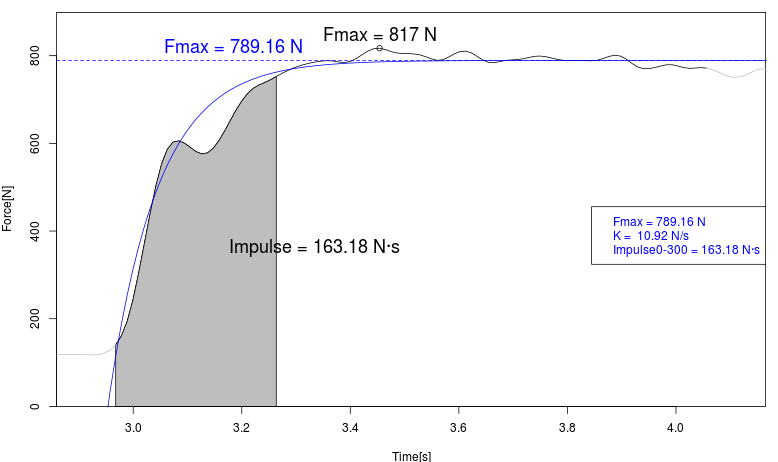Graphics with fitted data
Average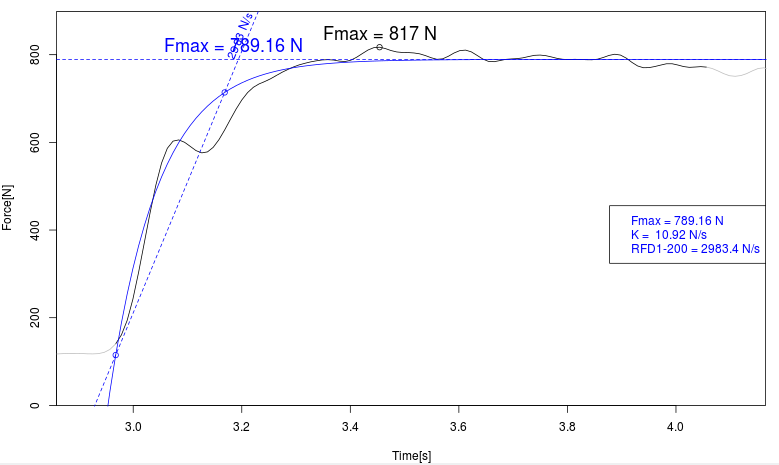Instant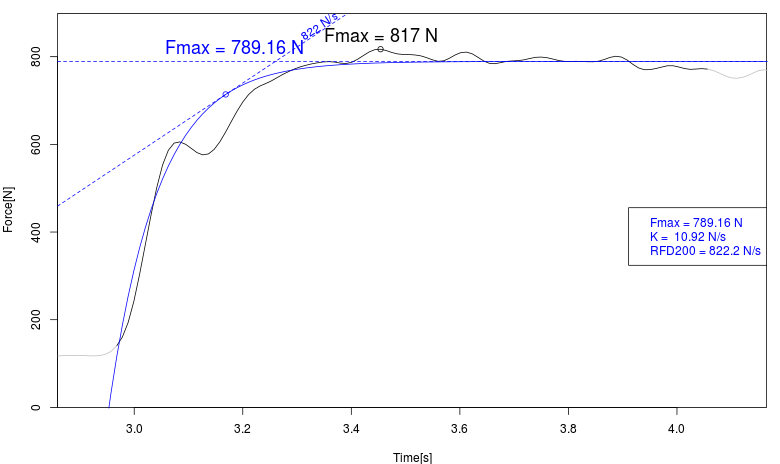% Fmax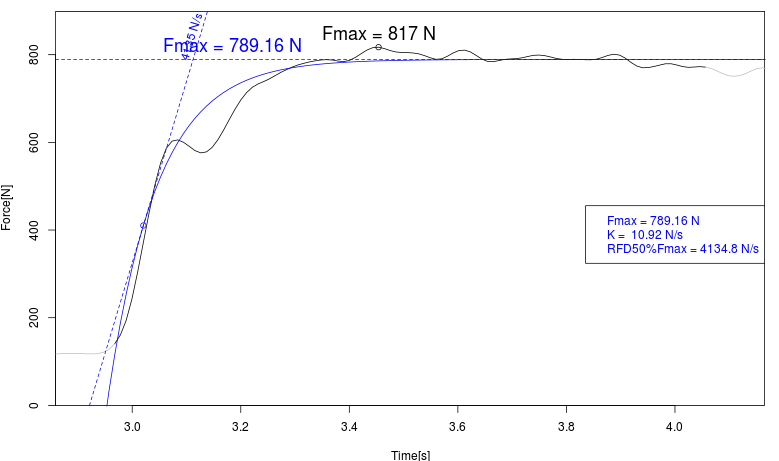Max RFD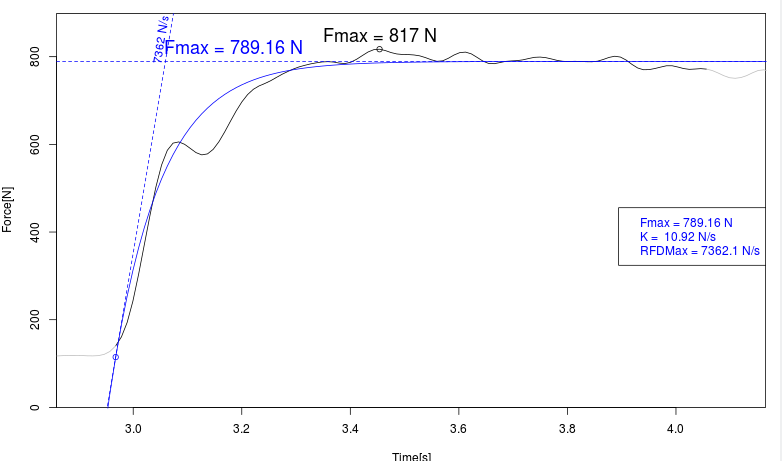Impulse